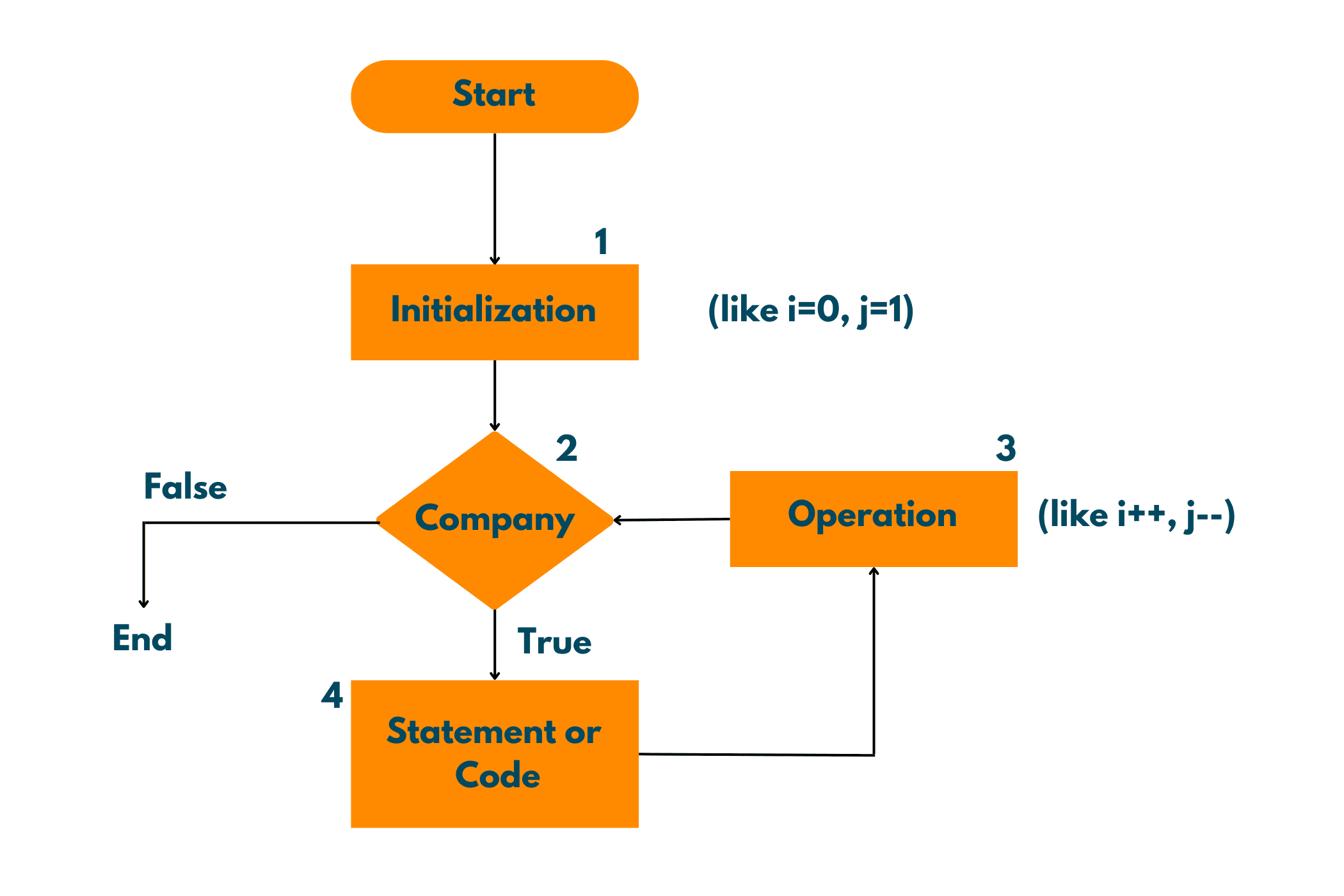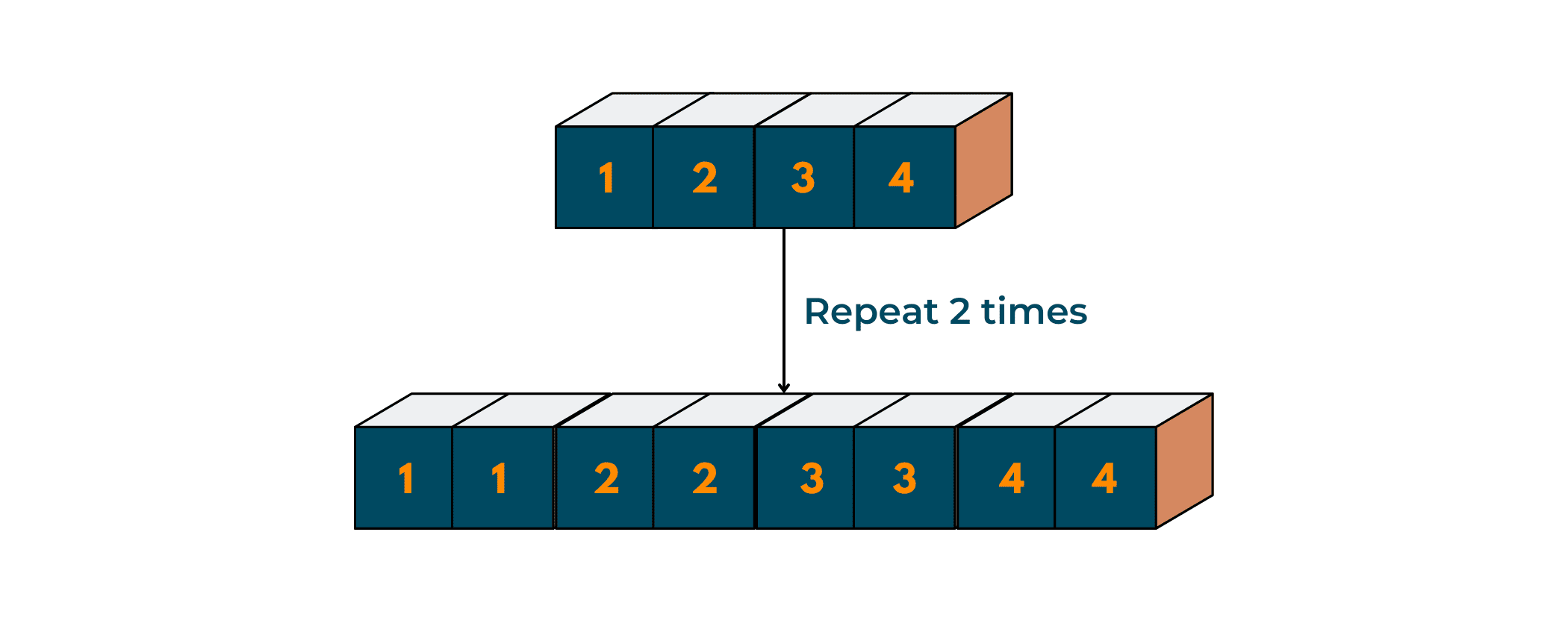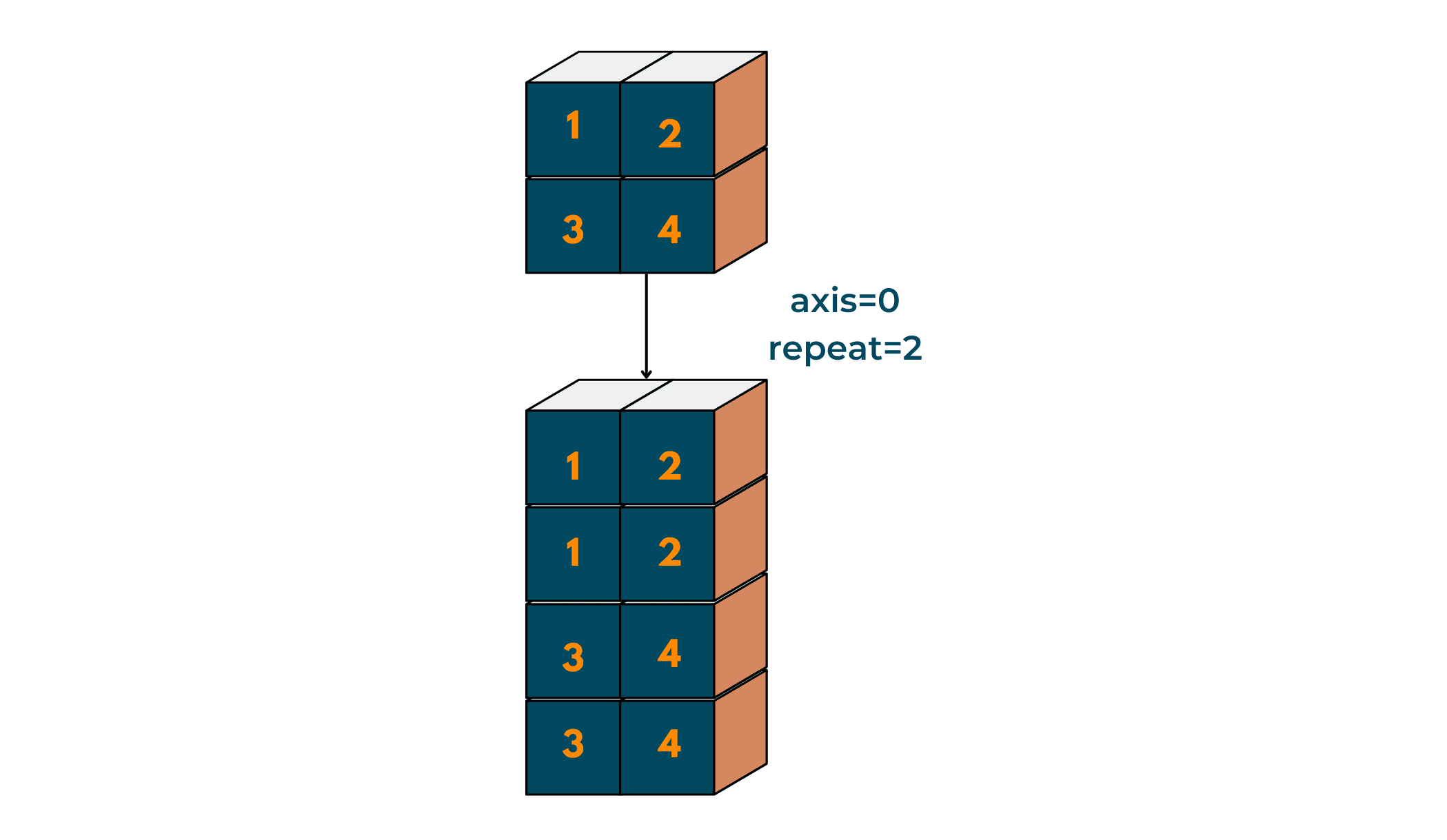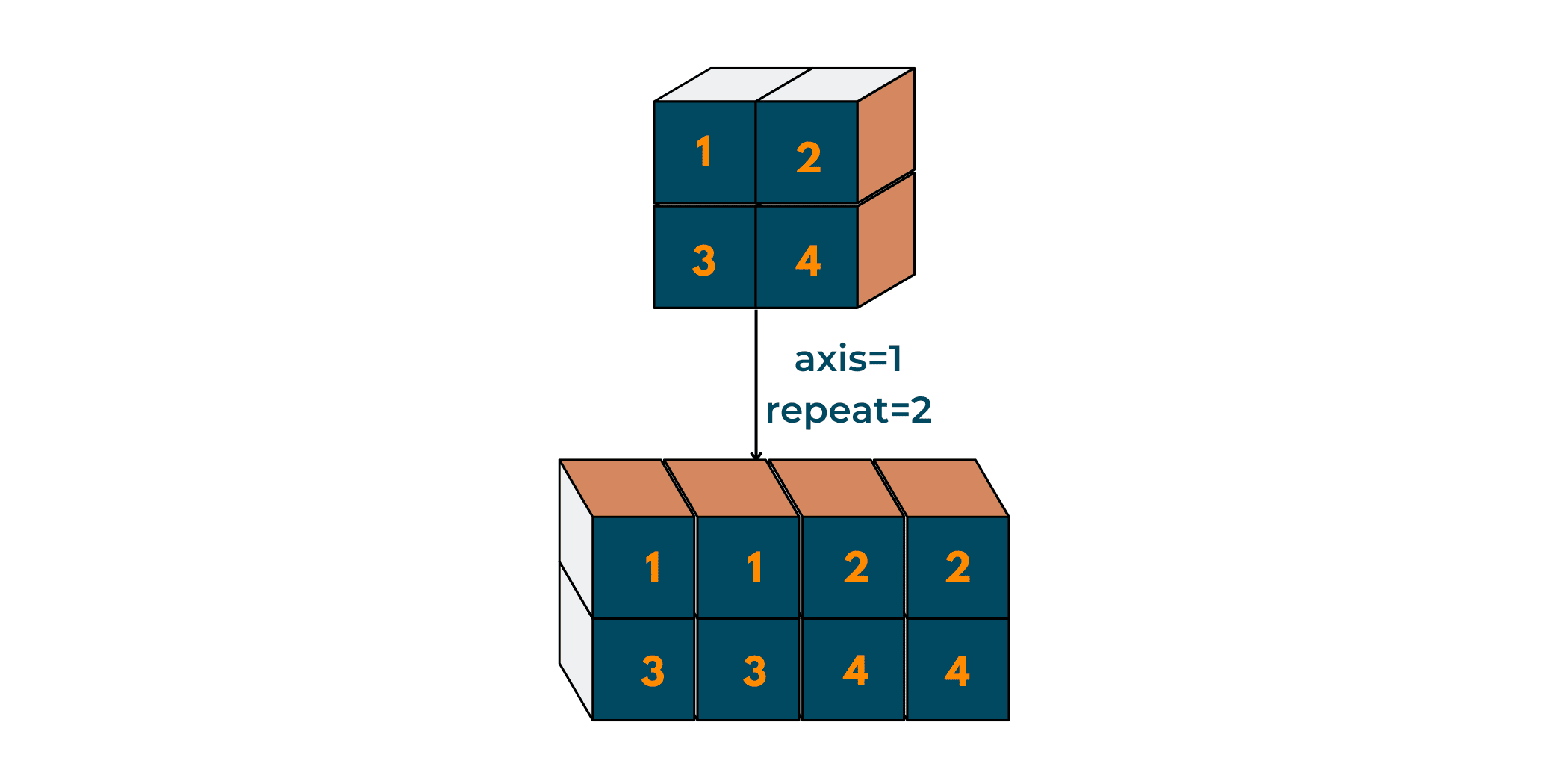# How to Repeat N times in Python? (& how to Iterate?)

We know that Python is an object-oriented programming language. It supports programming capabilities such as inheritance and polymorphism. "Code-reusability" is a key feature when working with programming languages.

This brings light to another topic: "Code Repetition in Python". You must be wondering what Code Repetition in Python is and what is it used for.

Well, to Repeat in Python refers to repeating a section of code in order to achieve a certain result. In simple terms, it is going over a piece of code a specific number of times. But why are we discussing Repeat in Python when DRY (don't repeat yourself) is at its peak now?

## Repeat in Python

As discussed above, repeat is basically going over a piece of code several times to achieve the desired output. This piece of code can be anything, some instructions, arithmetic functions, counter, or simple print operations.

You'll be surprised to know that the concept of Loops and Functions came into the picture because of Repeat in programming!

These have improved code readability while also reducing the number of lines of code. So, using this, we can also achieve optimization in Python.  Before moving on further, let's take a look at a few terms we're going to use below:

1. iterate: You might have often heard this word. The term 'iterate' goes synonymously with 'repeat'. So, 'iterating' is equivalent to 'repeating'.
2. n: Annotation to depict the number of times we expect to iterate the code.

## How to Iterate N times in Python?

Let's take a look at some methods to repeat in python programming Also, note that we're going to address many questions as we go down the article.

### 01) Using Loops

A loop is used to execute a set of instructions or a block of code several times without having to write it again. When dealing with loops, it is necessary to have a condition for the exit, or else you might end up with a never-ending (infinite) loop.

A basic loop includes the following operations:

1. Initialization
2. Condition check
3. Increment/decrement operations
4. Execution

Take a look at the image below to understand how a basic loop works:Now, that you've familiarized yourself with loops, let's move on to the loop offered by Python to iterate n times.

Using range() with For Loop

A for loop repeats a sequence until a condition is met. If you're familiar with other languages, you can also compare that the for loop offered by Python is more similar to the 'for-each' loop in other languages.

In Python, for loop is used to iterate over a sequence (like a list, a tuple, a dictionary, a set, or a string). A for loop in Python is explicitly called when the number of iterations is fixed.

A general for loop in Python looks like this:

for [variable_name] in [sequence_name] :

Let's take an example depicting the iteration over a sequence in Python:

```# Program: Iterate over a sequence

# a sequence
fruits = ["apple", "mango", "banana", "orange"]

# the for loop
for fruit in fruits:
print(fruit)

# here, the fruit is temporary variable while fruits is the list
```

Here, 'fruit' represents each element in the list of' fruits'.

Output:

```apple
mango
banana
orange
```

Now that we've looked into for loop in Python, let's move on to how for loop is used to repeat n times in Python.

range() function:

Python offers an in-built function that generates a sequence of numbers. The range() function, though used to produce a sequence of numbers, is also used with for loop as an exit statement (or to keep the count of iterations).

Syntax:

range ( start = 0, stop , step = 1)

The range function takes three parameters, namely start, stop and step.

1.  'Start' represents the number to begin the sequence with
2. 'stop' represents the number to end the sequence at. It never includes this number
3. 'step' represents the number to add (increment) with

For example, to generate a sequence like: 1, 2, 3, 4, 5, the parameters in the range() function will be:

start = 1

stop = 6 (since range() doesn't includes stop in range)

step = 1 (adds 1 to the previous number to generate the sequence)

By default, the values of start and step are 0 and 1 respectively. It is necessary to provide with a parameter as stop to the function.

Example:

```# Program: using range() function in Python

# Problem : get a sequence of natural numbers from 1 to 6
# Note that the number n is 1 more than the last digit
n = 7

for i in range(n):
# n is excluded
print(i, end=" ")
```

I've used 'end' to get the sequence in one line.

Output:

```0 1 2 3 4 5 6
```

The sequence obtained doesn't include the value of n. This was an example of repeating n times in Python.

Using while Loop

The while loop is another way to iterate n times in Python. It continues to execute until a given condition is met. It also requires an exit statement/condition to terminate the loop.

Example:

```# Program: Iterate n times in Python

# initialization
count = 1

# exit limit
n = 7

while count in range(n):
print(count)
# increment
count += 1
```

Output:

```1
2
3
4
5
6
```

Note that we've actually depicted how value is counted rather than repeating the same code n times.

### 02) Using Functions

As mentioned earlier, the need to repeat code gave rise to loops and functions. Functions are essentially a piece of code that you may reuse instead of writing them out several times. These increase code reusability and readability of the code.

A function is declared by:

def function_name (parameters):

{ statement }

Let's take a quick example of how to define a function in Python and how to call it in the program:

```# Program: Defining and calling functions

# defining a function
def repeat(parameter):
# use of * operator
print("Function called " * parameter)

# main body
n = 3
# calling the function
repeat(3)
```

Output:

```Function called Function called Function called
```

Although functions do not actually contribute to repeating/iterating n times in Python, they are a part of Code-repetition.

All of the above were user-defined methods, you must be wondering if there are any in-built functions in Python. Let's move to it!

## Is there a Repeat Function in Python?

There isn't any specific repeat function in the builtins module of Python. But Python offers modules like NumPy and pandas, which have their own repeat() function. The repeat() function is the function that will actually let you repeat the code n number of times in python.

### 01) Using itertools.repeat()

The itertools module provides a repeat() function in order to practice repeat in Python. In repeat(), we provide the data as well as the number of times the data will be repeated. Similar to the loops, it is necessary to specify the number, else the function won't be terminated and will end up in an indefinite loop.

Basic Syntax:

itertools.repeat( value, n )

The above syntax might not result in anything for you. The repeat() function actually repeats the value n times into a list, hence the obtained output needs to be stored in a list in order to be displayed. It works with-

print(list(itertools.repeat(value, n)))

Let's take an example to understand the use of itertools.repeat better:

```# Program: Using itertools.repeat() function to repeat n times in Python

# import repeat function from itertools module
from itertools import repeat

# defining n
n = 5

# define the value to be printed
s = "Repeat"

print(list(repeat(s, n)))
```

Output:

```['Repeat', 'Repeat', 'Repeat', 'Repeat', 'Repeat']
```

Note that the itertools module needs to be imported before calling Python's repeat() function.

### 02) Using numpy.repeat()

The NumPy module offers a repeat() function which 'repeats the elements of an array. Repeating the elements of an array intends that each element of the array is repeated n times and a new array is created with these repeated values.

Hence, with an array like -> array = [1, 2, 3, 4] ,

the result of repeating the array 1 time will be -> [1, 1, 2, 2, 3, 3, 4, 4]

Have a look at the below diagram for a better understanding:The numpy.repeat() function takes 3 parameters as input:

1.  arr -> Input array
2. n -> The number of repetitions to be made
3. axis -> This parameter gives the axis along which the elements are repeated. By default, it produces a flattened array. In general, any other value of axis except 0 doesn't work for 1-D array.

Syntax:

new_arr = numpy.repeat(arr, n, axis)

Let's take an example to understand the numpy.repeat() function:

```# Program: Using numpy.repeat() function to repeat n times in Python

# import numpy module
import numpy as np

# number of repetitions => n
n = 2

# array to perform repetition on
example = [1, 2,  3, 4]

# calling the repeat() function
new_arr = np.repeat(example, n)

print(new_arr)
```

Output:

```[1 1 2 2 3 3 4 4]
```

Now, let's try giving axis = 1 as a parameter:

```# Program: Using numpy.repeat() function to repeat n times in Python

# import numpy module
import numpy as np

# number of repetitions => n
n = 2

# array to perform repetition on
example = [1, 2,  3, 4]

# calling the repeat() function
# giving axis value = 1
new_arr = np.repeat(example, n, 1)

print(new_arr)
```

Output:

```Traceback (most recent call last):
File "main.py", line 14, in <module>
new_arr = np.repeat(example, n, 1)
File "<__array_function__ internals>", line 5, in repeat
File "/usr/lib/python3/dist-packages/numpy/core/fromnumeric.py", line 481, in repeat
return _wrapfunc(a, 'repeat', repeats, axis=axis)
File "/usr/lib/python3/dist-packages/numpy/core/fromnumeric.py", line 58, in _wrapfunc
return _wrapit(obj, method, *args, **kwds)
File "/usr/lib/python3/dist-packages/numpy/core/fromnumeric.py", line 47, in _wrapit
result = getattr(asarray(obj), method)(*args, **kwds)
numpy.AxisError: axis 1 is out of bounds for array of dimension 1
```

Note that an error is encountered while calling the numpy.repeat() function with axis=1. Now, that we've encountered an error in 1-D array, let's try to repeat() for a 2-Dimensional array with different axis values:

(i) For axis = 0

The obtained result will be like:```# Program: Using numpy.repeat() function to repeat n times in Python

# import numpy module
import numpy as np

# number of repetitions => n
n = 2

# 2-D array to perform repetition on
example = [[1, 2,] , [ 3, 4 ]]

# calling the repeat() function
# giving axis value = 1
new_arr = np.repeat(example, n, 0)

print(new_arr)
```

Output:

```[[1 2]
[1 2]
[3 4]
[3 4]]
```

(ii) For axis = 1

The obtained result will be like:```# Program: Using numpy.repeat() function to repeat n times in Python

# import numpy module
import numpy as np

# number of repetitions => n
n = 2

# 2-D array to perform repetition on
example = [[1, 2,] , [ 3, 4 ]]

# calling the repeat() function
# giving axis value = 1
new_arr = np.repeat(example, n, 1)

print(new_arr)
```

Output:

```[[1 1 2 2]
[3 3 4 4]]
```

Note the difference in the ways the outputs are presented, for the same array but different axis values.

Although we've seen the example to repeat n times in Python for arrays, numpy.repeat() also works on individual elements (like numbers). Let's take a look at that:

```# Program: Using numpy.repeat() function to repeat n times in Python

# import numpy module
import numpy as np

# number of repetitions => n
n = 5

# number to repeat
num = 0

# calling the repeat() function
new_arr = np.repeat(num, n)

print(new_arr)
```

Output:

```[0 0 0 0 0]
```

By default, the value of the axis is 0. It will return an error if we were to change the axis.

You can also try putting an array (like [1,2]) as repetitions in the function. Also, while you're at it, do try entering negative values for the axis. You'll be surprised by the result.

### 03) Using pandas.repeat() function

Pandas repeat() function returns output in a way similar to the NumPy module. Here, the repeat() function returns a new "Series" in which each element of the current Series is repeated a specified number of times in a row. This method requires the Series() function and repeat() function of the pandas module.

Note that Pandas Series is similar to a table column. It is a one-dimensional array that can hold any form of data.

Syntax:

Converting to series -> a = pandas.Series([list or data])

Calling for repeat() -> pandaSeries.repeat( n, axis )

Note that here the axis is set to None.

Let's take an example to understand repeat in Python using pandas module:

```# Program: Using pandas module to repeat n times in Python

# import pandas module
import pandas

# creating series
a = pandas.Series(['a', 'b', 'c'])
print('original series: ', a)

# calling the repeat() function
b = a.repeat(3)
print('repeated series: ', b)
```

Output:

```original series:  0    a
1    b
2    c
dtype: object
repeated series:  0    a
0    a
0    a
1    b
1    b
1    b
2    c
2    c
2    c
dtype: object
```

Hence, from the above examples, it is evident that there are modules that offer repeat() functions to repeat n times in Python.

So far we've discussed repeat() functions in Python. We've seen a few examples of how to implement these repeat functions on lists. Do you know that you can repeat a string n times in Python? Other than the above-listed methods, there are a few easy tricks that serve the purpose.

If you still have any doubts, solve your queries with our expert python tutors.

Let's check them out!

## Tricks to Repeat String n Times in Python

Have you ever tried using the operator '*' with a string?

It gave you an error, right?

But what if I were to tell you that you can use this * operator to repeat the string n times in Python? You won't believe me, right?

So, let's implement it.

The '*' operator is also known as the repetition operator. You can directly use the operator between the string to be printed and n, as string * n.

Example:

```# Program: To repeat a string n times in Python

# string
s = "Favtutor "

# number of times to be repeated
n = 3

print(s * 3)
```

Output:

```Favtutor Favtutor Favtutor
```

It worked right! Now if I were to tell you that you can also repeat a specific part of string n times in Python, then?

Let's check it out:

```# Program: To repeat a string n times in Python

# string
s = "Favtutor "

# number of times to be repeated
n = 3

# slicing the string
print(s[3:] * 3)
```

Output:

```tutor tutor tutor
```

You only need to select a part of the string using Slicing and then you're good to go.

## Conclusion

In this article, we've discussed how to repeat in Python and how it brought up the need for loops and functions. Further, we've learned about repeat() functions in python, offered by modules like itertools, pandas, and NumPy. Apart from them, we've also seen a trick to repeat strings n times in Python.

I suggest you implement the methods discussed above and also look out for more to compare and know the method which works better for you. Happy Coding!

### FavTutor - 24x7 Live Coding Help from Expert Tutors!